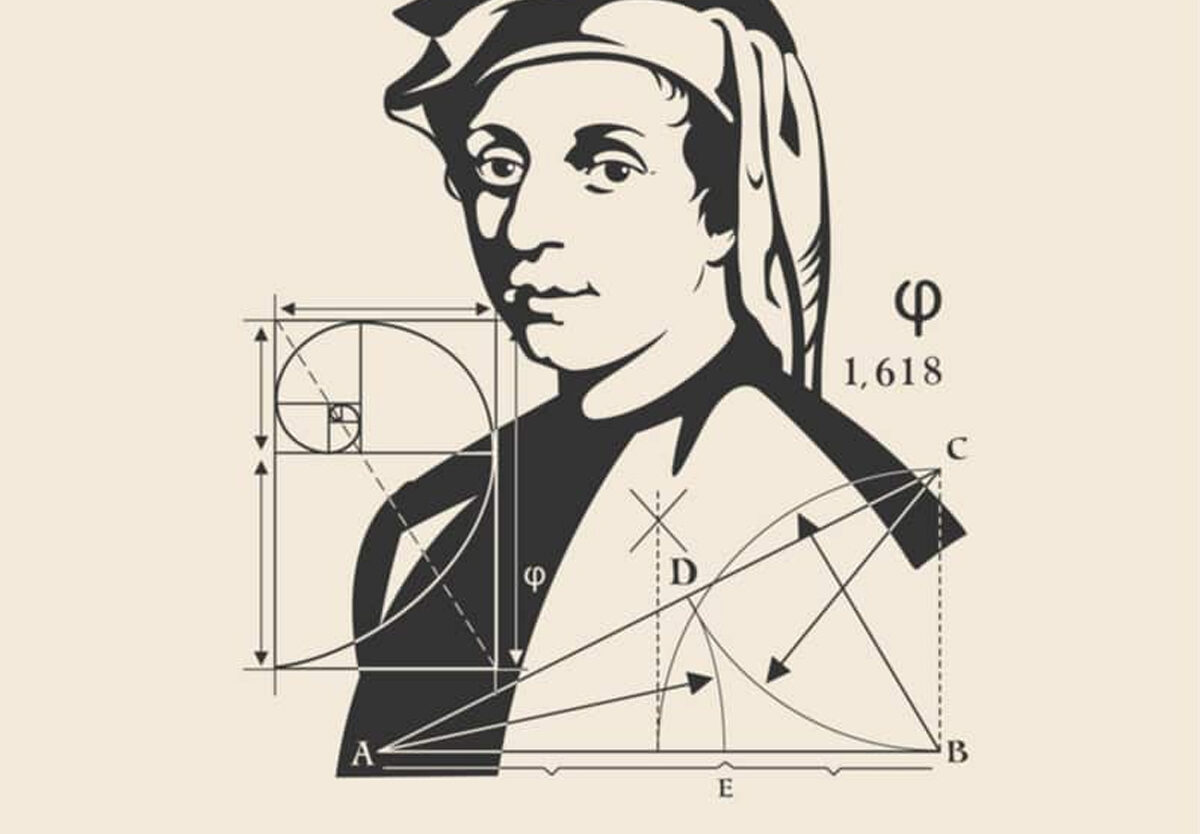##### Got an idea for a holiday? Send it to usSatNov 23

# Fibonacci Day – November 23, 2024

Also known as Leonardo of Pisa and Leonardo Fibonacci, Leonardo Bonacci invented a pattern of counting that continues to influence math and technology today. The pattern is made up of numbers that sum the previous two numbers before them — 1, 1, 2, 3, 5, 8, 13 — and so on. The sequence is used in computing, stock trading, and architecture and design.

Once we discovered the sequence, it started showing up everywhere. Nature is full of Fibonacci patterns, from DNA to hurricanes, leading some to dub the Fibonacci sequence “nature’s secret code.”

## History of Fibonacci Day

One of the most important mathematicians of the Middle Ages, Leonardo Bonacci — later known as Fibonacci, “the son of” Bonacci — invented a sequence of numbers that shows up constantly in nature, physics, and design.

Born to an Italian merchant, the young Leonardo traveled to North Africa with his father, where he was exposed to the Hindu-Arabic numeral system. The system, which includes zero and limits itself to 10 symbols, is much more agile and flexible compared to the unwieldy Roman numeral system. In 1202, Fibonacci published “Liber Abaci”, introducing Europe to the Hindu-Arabic system and his now-famous sequence.

Starting with 1, 1, 2, 3, 5, the Fibonacci sequence is created by adding up the two previous numbers to get the next one. Fibonacci’s original example for his sequence pondered the population growth of rabbits. If starting with one pair, and each month that pair bears a new pair, the number of rabbits will grow at a rate consistent with his pattern of numbers.

The Golden Ratio, a proportion associated with the Fibonacci sequence and also frequently found in nature, is roughly 1 to 1.6. This ratio shows up in the branching patterns of trees, the distribution of seeds in berries, the spiral arms of galaxies, and many more natural and human-engineered things.

Fibonacci Day celebrates this important mathematician and gives us an opportunity to marvel at the way math pervades everything around us. The Fibonacci sequence can be used to calculate the proportions of countless things on Earth and beyond, such as animals, plants, weather patterns, and even galaxies. Pause to observe your surroundings and you’ll start to notice the familiar spiral all around you.

## Fibonacci Day timeline

15th Century
Arabic Numerals Become the Norm

By the 15th century, the Hindu-Arabic numeral system largely replaces Roman numerals in Europe.

1240 - 1250
Fibonacci Dies

It's believed Leonardo Bonacci died sometime between 1240 and 1250 CE in Pisa, Italy.

1202
Arabic Numerals Introduced

Fibonacci's Liber Abaci(The Book of Calculation) introduces the Western world to the Hindu-Arabic numbering system we continue to use today, leading to the switch from Roman numerals and making complex calculations possible.

1170
Fibonacci Born

Leonardo Bonacci is born to an Italian merchant and customs official working in Algeria. It is in North Africa that Bonacci learns Hindu-Arabic numerals.

## Fibonacci Day FAQs

### Why is the Fibonacci sequence so important?

Fibonacci is remembered for two important contributions to Western mathematics, by helping spread the use of Hindu systems of writing numbers in Europe.

### Why is the Fibonacci sequence used in nature?

Thanks to one medieval man’s obsession with rabbits, we have a sequence of numbers that reflect various patterns found in nature. Each number is the sum of the previous two.

### Who is the greatest Fibonacci man?

Also known as Leonardo Bonacci, Leonardo of Pisa, or Leonardo Bigollo Pisano (‘Leonardo the Traveller from Pisa’), he was considered to be the most talented Western mathematician of the Middle Ages.

## How to Celebrate Fibonacci Day

1. ### Learn the sequence

How far in the sequence can you go? Remember, each number is the sum of the two numbers before it. Try reciting the sequence and see how far you get.

2. ### Look for the Golden Ratio in nature or household items

The Golden Ratio shows up in nature, architecture, and design. Find some examples around your house or neighborhood.

3. ### Make your own Fibonacci spiral

Try your hand drawing, sculpting, or baking the Fibonacci spiral into a design of your own.

## 5 Interesting Facts

1. ### Flower petals

Flower petals consistently come in Fibonacci numbers. Petals are arranged in a way that gives each one optimal sunlight and nutrients.

2. ### Seashells

The logarithmic spiral formed by the Golden Rectangle shows dramatically in the infinite spirals of seashells.

3. ### Spiral galaxies

Each arm of the Milky Way Galaxy is a logarithmic spiral.

4. ### Hurricanes

Like other weather phenomena, the spiral clouds of hurricanes follow a Fibonacci spiral.

5. ### Faces

Human and animal faces are often proportioned according to the Golden Ratio. Features that adhere most closely to these proportions are perceived as more pleasing or beautiful.

## Why We Love Fibonacci Day

1. ### The Fibonacci sequence is everywhere

The Fibonacci sequence and its associated visual forms, the Golden Rectangle and Golden Spiral, can be found in countless examples in nature, architecture, and engineering.

2. ### The Hindu-Arabic numerals revolutionized math

The introduction of the numerals we use today limited the number of digits, introduced the 0, and made it possible to make complex calculations.

3. ### Fibonacci numbers make computing possible

The Fibonacci sequence provides a model for programming algorithms and processing large amounts of data.

## Fibonacci Day dates

YearDateDay
2024November 23Saturday
2025November 23Sunday
2026November 23Monday
2027November 23Tuesday
2028November 23Thursday

## Holidays Straight to Your Inbox

Every day is a holiday!
Receive fresh holidays directly to your inbox.